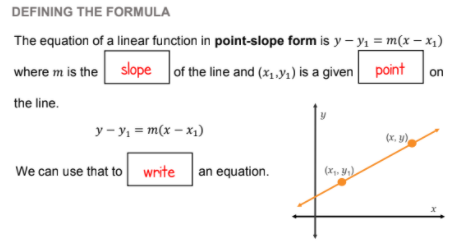# UNIT 2 LESSON 4 - DEVELOPING POINT SLOPE FORM

## WRITING THE LINEAR FUNCTION

What is the slope of the line?

What is the y-intercept?

Select all that apply
• A
• B
• C
• D
Because we do not have an integer as the y-intercept all of the time, we need to know how to get the equation for a line a different way. So how do we write equations for these graphs?

## POINT SLOPE FORMULATO USE POINT SLOPE FORMULA TO WRITE THE EQUATION FOR A LINE, ALL YOU NEED IS THE SLOPE AND ANY POINT!

## USING POINT SLOPE FORM PRACTICE

What is the slope of the line in the graph?

What would the equation be in point-slope form if you were using the point (-7,0)

Solving for y to get the equation in slope-intercept form would be...

What would the equation be in point-slope form if you were using the point (9,-4)

Solving for y to get the equation in slope-intercept form would be...

Does it matter which point you use to write your equation in point-slope form? Explain why or why not.